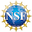ResearchTuesday, 22 August 2023 15:28

## Global well-posedness and refined regularity criterion for the uni-directional Euler-alignment system

Yatao Li, Qianyun Miao, Changhui Tan and Liutang Xue

Abstract

We investigate global solutions to the Euler-alignment system in d dimensions with unidirectional flows and strongly singular communication protocols $$\phi(x)=|x|^{-d+\alpha}$$ for $$\alpha\in(0,2)$$. Our paper establishes global regularity results in both the subcritical regime $$1<\alpha<2$$ and the critical regime $$\alpha=1$$. Notably, when $$\alpha=1$$, the system exhibits a critical scaling similar to the critical quasi-geostrophic equation. To achieve global well-posedness, we employ a novel method based on propagating the modulus of continuity. Our approach introduces the concept of simultaneously propagating multiple moduli of continuity, which allows us to effectively handle the system of two equations with critical scaling. Additionally, we improve the regularity criteria for solutions to this system in the supercritical regime $$0<\alpha<1$$.This work is supported by NSF grants DMS #2108264 and DMS #2238219

arXiv Preprint 2308.09609.